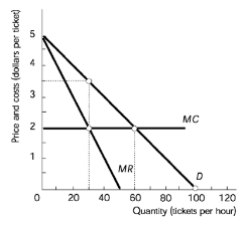Start typing, then use the up and down arrows to select an option from the list.## Microeconomics

Learn the toughest concepts covered in Microeconomics with step-by-step video tutorials and practice problems by world-class tutors

Monopoly

# Monopoly Efficiency and Deadweight Loss

Monopolies do not produce the efficient quantity.
1
concept

## Monopoly Efficiency and Deadweight Loss12m
Play a video:
2
Problem

An unregulated monopoly will sell:3
Problem

If the monopolist’s fixed cost is \$25, the monopoly’s total costs when maximizing profit is:4
Problem

If the monopolist’s fixed cost is \$25, the monopoly’s total economic profit when maximizing profit is:5
Problem

The deadweight loss created by the monopoly is: